# Active Band Pass Filter - Design Help

Joined Jan 24, 2011
9
Hi all,

I was hoping someone might be able to provide some guidance/point me in the right direction for the below problem:

The objective is to design an active bandpass with the following parameters:

Centre frequency Fc = 100kHz
Bandwith fH -fL = 10kHz

So far I have the following:

Given that the bandpass filter has a Centre or Resonant Frequency of 100kHz and a Bandwidth Frequency (BW) of 10kHz I have approximated the lower and upper cut off frequency at 95kHz and 105kHz respectively.

BW = fH - fL => 105k - 95kHz = 10kHz

Assuming a nominal Resistor value of 1K for both LPF and HPF circuits the capacitance values can then be obtained.

C1 = 1/2π.fL.R1 & C2 = 1/2π.fH.R2

The values of which are 1.67nF and 1.51nF respectively. These values are inputted into the below non inverting bandpass circuit. Circuit Gain selected to be around 10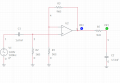The output waveform derived from this configuration is as per below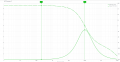As can be seen the output waveform is heavily attenuated and the calculated cut-off frequencies of 95k and 105k are thus not at 0.707 (-3db) at expected.

My question is how can this be configured so as to output the expected results at the appropriate frequencies?

Any advice guidance would be appreciated.

Last edited:

#### Papabravo

Joined Feb 24, 2006
20,386
I might wonder if the approach to the design of a bandpass filter, by cascading a high pass section, a gain stage, and a low pass section was flawed. The peak response in the @ericgibbs simulation is not at 100 kHz where it should be. Did the original problem state that you had to use two 1st order sections? They may not be able to achieve the desired roll off at 95 kHz and 105 kHz. This is a fairly narrow bandwidth and I'm guessing that a higher order filter will be required. The only way to be sure is to mathematically compute the required order to achieve the desired results.

#### crutschow

Joined Mar 14, 2008
32,903
That is not an active bandpass filter.
It is two passive RC filters with a gain-stage between them.
Active filters have feedback to improve the frequency response characteristics.
Look up Sallen-Key or Multiple-Feedback active filters to see how they are made.

Are there any requirements for the filter degree of rolloff?

Last edited:
•Ian0

#### LvW

Joined Jun 13, 2013
1,669
Hi all,
I was hoping someone might be able to provide some guidance/point me in the right direction for the below problem:

The objective is to design an active bandpass with the following parameters:

Centre frequency Fc = 100kHz
Bandwith fH -fL = 10kHz
........................

........................
Any advice guidance would be appreciated.
The quality factor of the desired bandpass is Q=100/10=10.
For bandpass circuits, this quality factor is identical to the so-called "pole-Q" which describes the position of the two poles of the 2nd-order function in the s-plane. For all values Qp>0.5 the poles are complex (a pole pair).
However, cascading a 1st-order lowpass with a 1st-order highpass allows only a pole position on the real axis of the s-plane (Qp<0.5).
Fazit: You must use either a pasive R-L-C resonant bandpass or an active second-order bandpass topology (Sallen-Key or multiple-feedback).

Joined Jan 24, 2011
9
Thanks everyone for their response.

There is no requirement for the degree roll off so I'll look at increasing the filter to 2 x 2pole filters to increase the roll off rate. Below is a working copy of proposed amendment. The gain values have been obtained to achieve a Butterworth response as per below table.

I think the issue appears to be the small bandwidth between the 2 cut-off points as this is very close to the centre frequency.

The RC values have been retained from the original circuit, based on earlier calculations however probably need to verify again.

Hoping this approach is more in the right direction.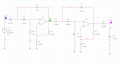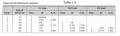Thanks

#### Ramussons

Joined May 3, 2013
1,354
What you are constructing is a Passive LPF and HPF with an amplifier in between.

Joined Jan 24, 2011
9
What you are constructing is a Passive LPF and HPF with an amplifier in between.
There is now feedback via the amplifier to input side of the circuit. Does this not therefore class it as Active?

#### Ramussons

Joined May 3, 2013
1,354
There is now feedback via the amplifier to input side of the circuit. Does this not therefore class it as Active?
Yes, it is active.
Knock off R11 and C8 which is passive.

(My post #7 uploaded too late.)

Joined Jan 24, 2011
9
Yes, it is active.
Knock off R11 and C8 which is passive.

(My post #7 uploaded too late.)
Ah yes, thanks.

Left that on the circuit in error

#### MrAl

Joined Jun 17, 2014
10,602
Hi all,

I was hoping someone might be able to provide some guidance/point me in the right direction for the below problem:

The objective is to design an active bandpass with the following parameters:

Centre frequency Fc = 100kHz
Bandwith fH -fL = 10kHz

So far I have the following:

Given that the bandpass filter has a Centre or Resonant Frequency of 100kHz and a Bandwidth Frequency (BW) of 10kHz I have approximated the lower and upper cut off frequency at 95kHz and 105kHz respectively.

BW = fH - fL => 105k - 95kHz = 10kHz

Assuming a nominal Resistor value of 1K for both LPF and HPF circuits the capacitance values can then be obtained.

C1 = 1/2π.fL.R1 & C2 = 1/2π.fH.R2

The values of which are 1.67nF and 1.51nF respectively. These values are inputted into the below non inverting bandpass circuit. Circuit Gain selected to be around 10

View attachment 263501

The output waveform derived from this configuration is as per below

View attachment 263502

As can be seen the output waveform is heavily attenuated and the calculated cut-off frequencies of 95k and 105k are thus not at 0.707 (-3db) at expected.

My question is how can this be configured so as to output the expected results at the appropriate frequencies?

Any advice guidance would be appreciated.
Hello,

As you can see by the output that's not too bad of a way of doing it really as long as you have the high pass section ahead of the low pass section. The nice thing about it is that the step response is clean.

As to your amplitudes being not what you expected, that's simply because two filters in isolated series like that do not produce 0.7071 they produce a lower value than that, maybe around 0.5. That means your amplification factor has to be somewhere around 2 to get that 0.7071 output value.
Your gain right now looks like it is 10, which would be too high.

The values for the R's and C's can be derived analytically from a network analysis of the transfer function. It's not especially difficult if you want to go through this. You can get exact output levels that way, but even simpler you can get very close approximate output levels by assuming that the lower frequency is 95/100 times the center frequency and the upper frequency is 105/100 times the center frequency. That's not exact BTW but it comes out very close. If you want to look at this, left me know.

Joined Jan 24, 2011
9
Hello,

As you can see by the output that's not too bad of a way of doing it really as long as you have the high pass section ahead of the low pass section. The nice thing about it is that the step response is clean.

As to your amplitudes being not what you expected, that's simply because two filters in isolated series like that do not produce 0.7071 they produce a lower value than that, maybe around 0.5. That means your amplification factor has to be somewhere around 2 to get that 0.7071 output value.
Your gain right now looks like it is 10, which would be too high.

The values for the R's and C's can be derived analytically from a network analysis of the transfer function. It's not especially difficult if you want to go through this. You can get exact output levels that way, but even simpler you can get very close approximate output levels by assuming that the lower frequency is 95/100 times the center frequency and the upper frequency is 105/100 times the center frequency. That's not exact BTW but it comes out very close. If you want to look at this, left me know.
Hello,

Yes, would be much appreciated if you provide some guidance with regard to performing a network analysis of the transfer function., Thanks

By the ways after some advice received from this forum I have started to investigate the option of using 2 pole Sallen-key active filter as below: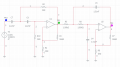I have used the same RC values from the original circuit. Prior to cascading these circuits together I performed an AC sweep for these as separate circuits (each with it's own supply) and the below response was obtained. Green for the high pass and purple for the low. The results seem to be correct but the issue appears to be the extremely narrow bandwidth before cut-off. It's also clear that Opamp does not function correctly at high frequencies, which as I understand relates to the unity Gain bandwidth of the opamp however I dont think in this case this is a problem as the upper cut off is 105K.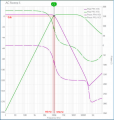When the circuits are cascaded together the results obtained are shown. The same problem arises in that the Centre frequency may be correct but the cut off frequencies do not occur at -3db. Cut off occurs at more or less the same amplitude as the Centre frequency with only a negligible fall .

I'm not sure how to overcome this problem. Do I need an even greater roll? Does it make any difference if I change the capacitance values as opposed to using same values for each circuit?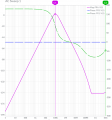Thanks

#### LvW

Joined Jun 13, 2013
1,669
Hello,

By the ways after some advice received from this forum I have started to investigate the option of using 2 pole Sallen-key active filter as below:
:::::::::::::::::::::::::::::::::::::::::::::::::::::::::::::::::
When the circuits are cascaded together the results obtained are shown. The same problem arises in that the Centre frequency may be correct but the cut off frequencies do not occur at -3db. Cut off occurs at more or less the same amplitude as the Centre frequency with only a negligible fall .

I'm not sure how to overcome this problem. Do I need an even greater roll? Does it make any difference if I change the capacitance values as opposed to using same values for each circuit?
Thanks
No surprise: Connecting a 2nd-order highpass with a 2nd-order lowpass (each with its own 3dB cutoff) you must not expect that the resulting bandpass will have a 3dB bandwidth according to these two 3dB frequencies. This will be the case only if both 3dB corners are suffieciently "far" to each other (quality factor below unity). However, in your case (with Q=10), the separate Bode diagrams show how and where both magnitude functions overlap in the critical region.
For such a 4th-order bandpass (Q=10) you have no other choise than (a) to use a suitable filter design program or (b) make a design by yourself starting with a corresponding lowpass function and then apply the lowpass-to-bandpass transformation.

#### crutschow

Joined Mar 14, 2008
32,903
You could improve the response some if you use a 3-pole Sallen-Key high-pass and low-pass filter.
The design of these is shown here.

Joined Jan 24, 2011
9
You could improve the response some if you use a 3-pole Sallen-Key high-pass and low-pass filter.
The design of these is shown here.
Thanks crutschow,

I'm assuming the improvement is given from the steeper roll of rate of the filters?

Joined Jan 24, 2011
9
No surprise: Connecting a 2nd-order highpass with a 2nd-order lowpass (each with its own 3dB cutoff) you must not expect that the resulting bandpass will have a 3dB bandwidth according to these two 3dB frequencies. This will be the case only if both 3dB corners are suffieciently "far" to each other (quality factor below unity). However, in your case (with Q=10), the separate Bode diagrams show how and where both magnitude functions overlap in the critical region.
For such a 4th-order bandpass (Q=10) you have no other choise than (a) to use a suitable filter design program or (b) make a design by yourself starting with a corresponding lowpass function and then apply the lowpass-to-bandpass transformation.
Thanks LvW,

I'm not clear on the below statement.

"b) make a design by yourself starting with a corresponding lowpass function and then apply the lowpass-to-bandpass transformation"

This relates to the below transformation function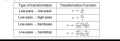Thanks again

#### Papabravo

Joined Feb 24, 2006
20,386
It tells you how to transform the transfer function of a low-pass filter into a transfer function of another type by a simple substitution. The first transformation tells you how to transform a low-pass with a normalized corner frequency of ω = 1 rad/sec into a low-pass filter for any other frequency. Similarly, it tells you how to transform a low-pass filter into a high-pass filter, with a simple substitution. The algebra of the low-pass to bandpass transformation is a bit messy, but well worth the effort.

#### crutschow

Joined Mar 14, 2008
32,903
I'm assuming the improvement is given from the steeper roll of rate of the filters?
Yes.

#### bertus

Joined Apr 5, 2008
22,217
Hello,

Have a look at section 16.5 of the attached PDF.

Bertus

#### Attachments

• 314.4 KB Views: 7

#### MrAl

Joined Jun 17, 2014
10,602
Hello,

Yes, would be much appreciated if you provide some guidance with regard to performing a network analysis of the transfer function., Thanks

By the ways after some advice received from this forum I have started to investigate the option of using 2 pole Sallen-key active filter as below:
View attachment 263854

I have used the same RC values from the original circuit. Prior to cascading these circuits together I performed an AC sweep for these as separate circuits (each with it's own supply) and the below response was obtained. Green for the high pass and purple for the low. The results seem to be correct but the issue appears to be the extremely narrow bandwidth before cut-off. It's also clear that Opamp does not function correctly at high frequencies, which as I understand relates to the unity Gain bandwidth of the opamp however I dont think in this case this is a problem as the upper cut off is 105K.

View attachment 263855

When the circuits are cascaded together the results obtained are shown. The same problem arises in that the Centre frequency may be correct but the cut off frequencies do not occur at -3db. Cut off occurs at more or less the same amplitude as the Centre frequency with only a negligible fall .

I'm not sure how to overcome this problem. Do I need an even greater roll? Does it make any difference if I change the capacitance values as opposed to using same values for each circuit?

View attachment 263856

Thanks
When you cascade filters where each section has very low output impedance the two transfer functions are multiplied together, so it is very simple to think about. If you have two filters with transfer functions Ts1 and Ts2, that means:
Ts=Ts1*Ts2

Now if each of the two have a response of 0.7 at the mid point frequency (really anywhere though), then the amplitude at that frequency is:
A=A1*A2=0.7*0.7=0.49

From that you can see that the total response went down quite a bit. You would need to add amplification to that setup to get an output of 0.7 again:
G=0.7/0.49=1.42857

and then the output amplitude will be 0.7 as desired.

For a quick rundown of the network analysis, if you know how to solve resistor problems such as with Nodal Analysis then you can replace the capacitors with their impedance z=1/(s*C) and then proceed as before.
For a high pass RC section this would look like this:
R/(R+1/(s*C)

and so it's just a voltage divider circuit that is frequency dependent.